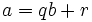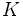# Euclidean domain

This article defines a property of integral domains, viz., a property that, given any integral domain, is either true or false for that.
View other properties of integral domains | View all properties of commutative unital rings
VIEW RELATED: Commutative unital ring property implications | Commutative unital ring property non-implications |Commutative unital ring metaproperty satisfactions | Commutative unital ring metaproperty dissatisfactions | Commutative unital ring property satisfactions | Commutative unital ring property dissatisfactions

## Definition

### Symbol-free definition

An integral domain is said to be Euclidean if it admits a Euclidean norm.

### Definition with symbols

An integral domain$R$ is termed a Euclidean domain if there exists a function$N$ from the set of nonzero elements of$R$ to the set of nonnegative integers satisfying the following properties:

•$N(x) = 0$ if and only if$x$ is a unit
• Given nonzero$a$ and$b$ in$R$, there exist$q$ and$r$ such that$a = qb + r$ and either$r = 0$ or$N(r) < N(b)$.

We call$a$ the dividend,$b$ the divisor,$q$ the quotient and$r$ the remainder.

Such a function$N$ is called a Euclidean norm on$R$.

### Caveats

• The definition of Euclidean norm does not require the ring to be an integral domain. A commutative unital ring that admits a Euclidean norm is termed a Euclidean ring.
• The definition of Euclidean domain does not require that$q$ and$r$ be uniquely determined from$a$ and$b$. If$q$ and$r$ are uniquely determined from$a$ and$b$, the integral domain is termed a uniquely Euclidean domain.

## Examples

### Other examples

• The ring of Gaussian integers$\mathbb{Z}[i]$ is a Euclidean domain with Euclidean norm equal to the norm in the sense of a quadratic integer ring. For full proof, refer: Ring of Gaussian integers is norm-Euclidean
• A quadratic integer ring, or more generally, a ring of integers in a number field, is termed norm-Euclidean ring of integers in a number field if it is Euclidean with respect to the algebraic norm. Since there is a correspondence between number fields and their rings of integers, we often abuse language and say that the number field itself is norm-Euclidean.
• Any discrete valuation ring is a Euclidean domain where the norm of an element is given by the largest power of the irreducible that divides it. For instance, the formal power series ring over a field is a Euclidean domain, where the norm of a formal power series is the smallest$n$ for which the coefficient of$x^n$ that is nonzero.

### Pathological examples

On a field, any norm function is Euclidean. This is because we can always choose a quotient so that the remainder is zero.

## Relation with other properties

### Stronger properties

Property Meaning Proof of implication Proof of strictness (reverse implication failure) Intermediate notions
uniquely Euclidean domain there is a Euclidean norm for which Euclidean division is unique. click here
Polynomial ring over a field it can be written as the polynomial ring$K[x]$ for a field$K$. click here

### Weaker properties

Property Meaning Proof of implication Proof of strictness (reverse implication failure) Intermediate notions
principal ideal domain integral domain that is a principal ideal ring Euclidean implies PID PID not implies Euclidean click here
Bezout domain integral domain in which every finitely generated ideal is principal click here
Noetherian domain integral domain and every ideal is finitely generated click here

### Properties of Euclidean norms

Euclidean norms can in general be very weirdly behaved, but some Euclidean norms are good. For a complete list of properties of Euclidean norms (i.e., properties against which a given Euclidean norm can be tested), refer:

Here are some important properties that most typical Euclidean norms satisfy:

## Metaproperties

### Polynomial-closedness

This property of commutative unital rings is not closed under passing to the polynomial ring

The polynomial ring over a Euclidean domain need not be a Euclidean domain. One example is the polynomial ring with integer coefficients, which is not a Euclidean domain; another example is the polynomial ring in two variables over a field (which can be viewed as the polynomial ring in one variable, over the polynomial ring over a field).